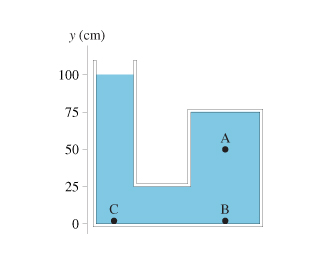# What is the pressure difference between points A and C? PHYSICS!?

+1 vote

edited

What is the pressure difference between points A and C? PHYSICS!?

The container shown in the figure(Figure 1) is filled with oil. It is open to the atmosphere on the left.

Figure 1:Part A

What is the pressure at point A?

Part B

What is the pressure difference between points A and B?

Part C

What is the pressure difference between points A and C?

by

The ol type is not specified. Density of oil varies from less than 800 kg / m^3 to more than 920 kg/m^3

We can only assume a 'mean' density of 850 kg / m^3

Part A:

Point A is 50cm below liquid surface.

P = h. d. g = 0.5 x 850 x 9.81 = 4169 Pa

Part B: A and B are 50 cms apart.

So, pressure difference is again 4169 Pa

Part C : A and C are 50 cms apart.

So, pressure difference is once again 4169 Pa

Lorem ipsum dolor sit amet, consectetur adipiscing elit, sed do eiusmod tempor incididunt ut labore et dolore magna aliqua. Ut enim ad minim veniam, quis nostrud exercitation ullamco laboris nisi ut aliquip ex ea commodo consequat. Duis aute irure dolor in reprehenderit in voluptate velit esse cillum dolore eu fugiat.
by

Assuming you have the same book as me, the density of oil is 900 kg/m^3

Relevant equations:

P=Po+hdg

Where P is the pressure you are trying to find, Po is any known pressure, h is the difference in height in meters, d is the density, and g is the acceleration of gravity.

Known:

g = 9.8m/s^2

d = 900kg/m^3

Po = 101300 Pa (atmospheric pressure)

Since the left side of the tank is open to the atmosphere, the pressure at the top left of the tank is going to be equal to the pressure of the atmosphere. This is why we use the atmospheric pressure as our Po.

Calculations:

Pa = Po + hdg

= 101300 + (1 - .5)(900)(9.8)

= 105710 Pa

= 106 kPa (using 3 significant figures)

Since B and C are the same height, the answers will be the same

Pb = Pc = Po + hdg

= 101300 + (1 - 0)(900)(9.8)

= 110120 Pa

Pb - Pa = 110120 - 105710

= 4410 Pa

= 4.4 kPa (to two significant figures)

Pc - Pa = Pb - Pa

= 4.4 kPa

Lorem ipsum dolor sit amet, consectetur adipiscing elit, sed do eiusmod tempor incididunt ut labore et dolore magna aliqua. Ut enim ad minim veniam, quis nostrud exercitation ullamco laboris nisi ut aliquip ex ea commodo consequat. Duis aute irure dolor in reprehenderit in voluptate velit esse cillum dolore eu fugiat.
by

Po + (Density)gd

= 1.013*10^5+900*9.8*.5

=1.057 then subtract the gauge pressure of 101.3kPa

this should roughly give you

4400 Pa

that is EXACTLY the right Pa

Lorem ipsum dolor sit amet, consectetur adipiscing elit, sed do eiusmod tempor incididunt ut labore et dolore magna aliqua. Ut enim ad minim veniam, quis nostrud exercitation ullamco laboris nisi ut aliquip ex ea commodo consequat. Duis aute irure dolor in reprehenderit in voluptate velit esse cillum dolore eu fugiat.

+1 vote